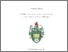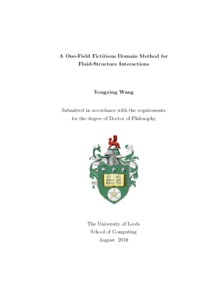# A One-Field Fictitious Domain Method for Fluid-Structure Interactions

Wang, Yongxing (2018) A One-Field Fictitious Domain Method for Fluid-Structure Interactions. PhD thesis, University of Leeds.Preview
Text
Wang_Y_Computing_PhD_2018.PDF - Final eThesis - complete (pdf)
We present a one-field fictitious domain method (FDM) for simulation of general fluid-structure interactions (FSI). "One-field" means only one velocity field is solved in the whole (fluid and solid) domain based upon the finite element interpolation. The proposed method has the same generality and robustness as the FDM with a distributed Lagrange multiplier (DLM): both of them solve the fluid equations and solid equations as one system. However the one-field FDM only needs to solve for one velocity field while the FDM/DLM usually solves for fluid velocity, solid displacement and Lagrange multiplier. The proposed one-field FDM also has similar features with immersed finite element methods (IFEM): the explicit or implicit IFEM places all the solid information in a FSI force term which is arranged on the right-hand side of the fluid equations. The one-field FDM assembles the solid equations and implicitly includes them with the fluid equations. What we achieve is theoretically equivalent to an implicit IFEM but avoiding convergence problems, and a wide range of solid parameters can be considered in this scheme. In short, the one-field FDM combines the FDM/DLM advantage of robustness and the IFEM advantage of efficiency. In this thesis, we present a thorough review, summary and categorization of the existing finite element methods for FSI problems. The finite element weak formulation of the one-field FDM and discretization in time and space are introduced, followed by a stability analysis by energy estimate. The proposed scheme is first implemented in implicit form, followed by numerical validation for the property of non-increasing energy under the conditions of $\rho^f\le\rho^s$ (densities of the fluid and solid respectively) and $\nu^f\le\nu^s$ (viscosities of the fluid and solid respectively), and numerical tests for stability under the conditions of $\rho^f>\rho^s$ and/or $\nu^f>\nu^s$. The proposed scheme is then implemented based upon three explicit splitting schemes: 2-step splitting, 3-step splitting and 4-step splitting scheme. The fully coupled implicit FSI system is decoupled into subproblems step by step, which can be effectively solved. The pros and cons of these splitting schemes are analysed followed by a selection of numerical tests in order to illustrate the capabilities and range of applicability of the proposed one-field FDM scheme. The thesis concludes with a presentation of some topics and open problems that may be worthy of further investigation.